Next: Existence of a point Up: (Usual) affine schemes Previous: ring homomorphism and spectrum

## localization of a commutative ring

.

DEFINITION 1.6   Let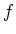be an element of a commutative ring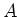. Then we define the localization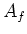ofwith respect toas a ring defined by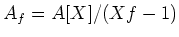where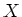is a indeterminate.

In the ring, the residue class ofplays the role of the inverse of. Therefore, we may write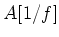instead ofif there is no confusion.

One may define localization in much more general situation. The reader is advised to read standard books on commutative algebras.

LEMMA 1.7   Letbe an element of a commutative ring. Then there is a canonically defined homeomorphism betweenand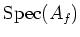. (It is usual to identify these two via this homeomorphism.)

PROOF.. Let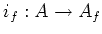be the natural homomorphism. We have already seen that we have a continuous map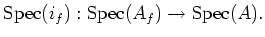We need to show that it is injective, and that it gives a homeomorphism betweenand.

Let us do this by considering representations.

1.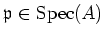corresponds to a representation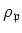.

2.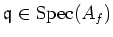corresponds to a representation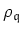.

3.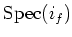corresponds to a restriction map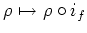.

Now, for any,extends toif and only if the image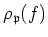ofis invertible, that means,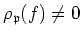. In such a case, the extension is unique. (We recall the fact that the inverse of an element of a field is unique.)

It is easy to prove thatis a homeomorphism.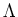Letbe a ring. Let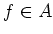. It is important to note that each element ofis written as a fraction''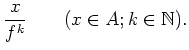One may introduceas a set of such formal fractions which satisfy ordinary computation rules. In precise, we have the following Lemma.

LEMMA 1.8   Letbe a ring,be its element. Let us consider the following set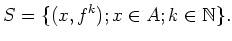We introduce on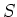the following equivalence law.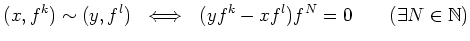Then we may obtain a ring structure on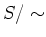by introducing the following sum and product.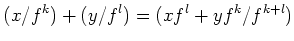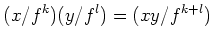where we have denoted by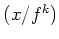the equivalence class of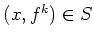.

COROLLARY 1.9   Letbe a ring,be its element. Then we have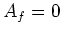if and only ifis nilpotent.

Likewise, for any-module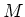, we may define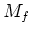as a set of formal fractions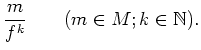which satisfy certain computation rules.

SubsectionsNext: Existence of a point Up: (Usual) affine schemes Previous: ring homomorphism and spectrum
2007-12-11